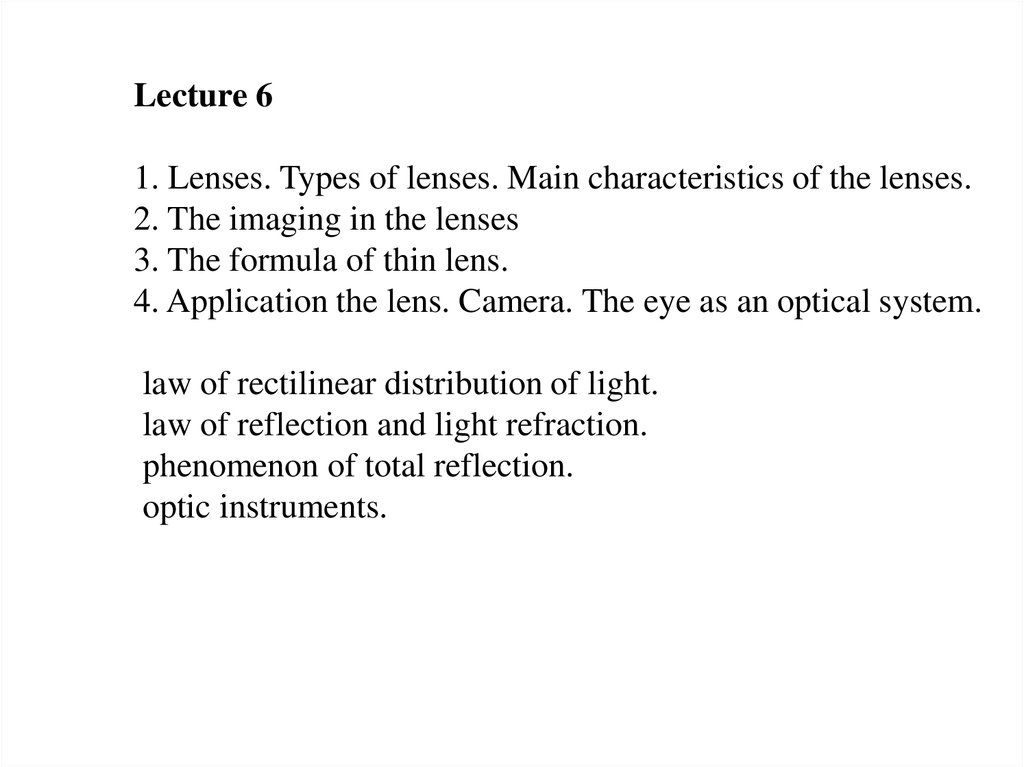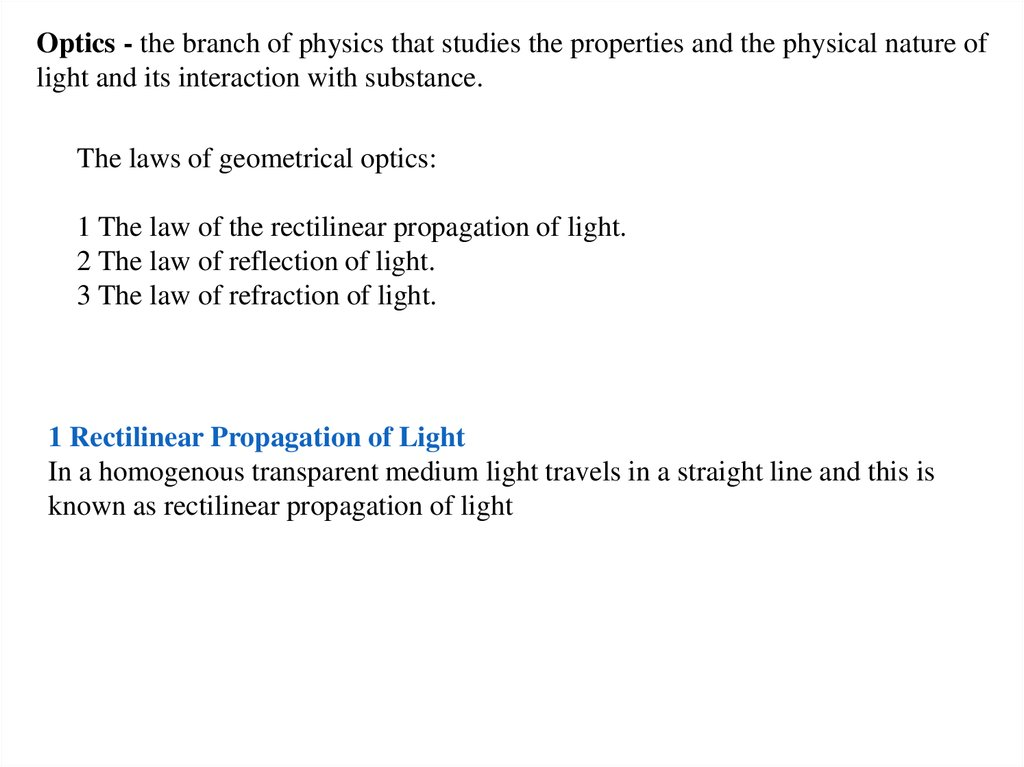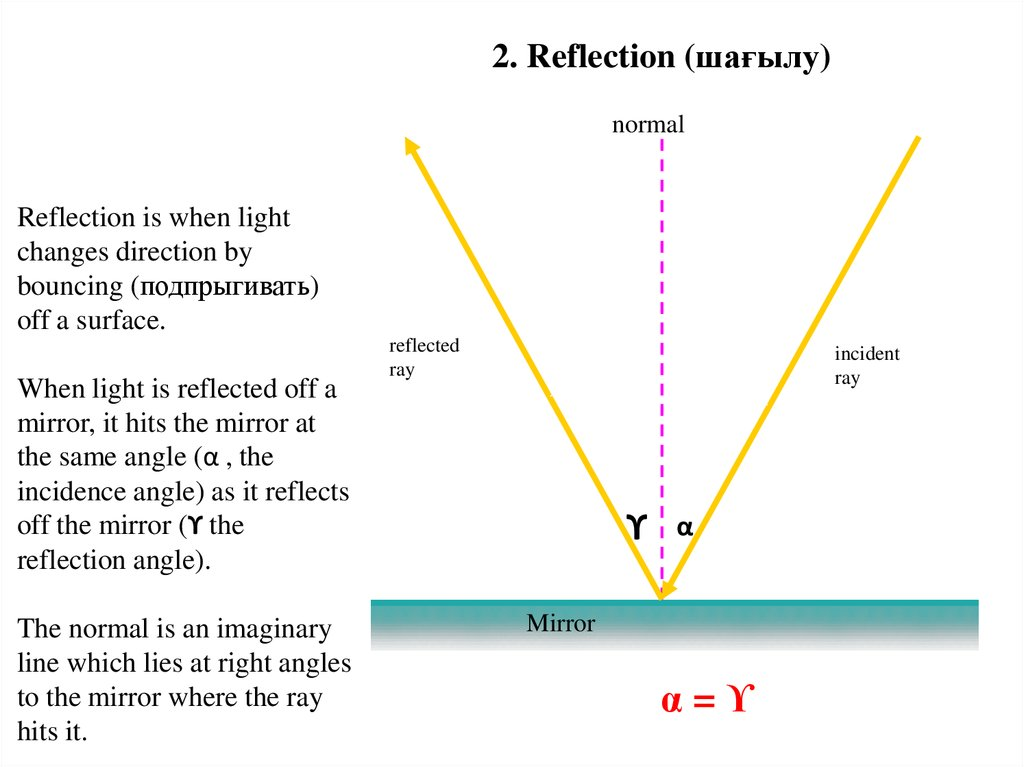# Lenses. Types of lenses. Main characteristics of the lenses

## 1.

Lecture 6
1. Lenses. Types of lenses. Main characteristics of the lenses.
2. The imaging in the lenses
3. The formula of thin lens.
4. Application the lens. Camera. The eye as an optical system.
law of rectilinear distribution of light.
law of reflection and light refraction.
phenomenon of total reflection.
optic instruments.

## 2.

Optics - the branch of physics that studies the properties and the physical nature of
light and its interaction with substance.
The laws of geometrical optics:
1 The law of the rectilinear propagation of light.
2 The law of reflection of light.
3 The law of refraction of light.
1 Rectilinear Propagation of Light
In a homogenous transparent medium light travels in a straight line and this is
known as rectilinear propagation of light

## 3.

2. Reflection (шағылу)
normal
Reflection is when light
changes direction by
bouncing (подпрыгивать)
off a surface.
When light is reflected off a
mirror, it hits the mirror at
the same angle (α , the
incidence angle) as it reflects
off the mirror (ϒ the
reflection angle).
The normal is an imaginary
line which lies at right angles
to the mirror where the ray
hits it.
reflected
ray
incident
ray
ϒ
α
Mirror
α=ϒ

## 4.

3. Refraction (сыну)
Refraction is when light bends as it passes
from one medium into another.
When light traveling through air passes
into the glass block it is refracted towards
the normal.
normal
air
α
glass
block
β
When light passes back out of the glass
into the air, it is refracted away from the
normal.
β
α
normal
air

## 5.

Lenses - a piece of transparent substance, usually glass, having two opposite
surfaces either both curved or one curved and one plane, used in an optical
device in changing the convergence of light rays, as for magnification, or in
correcting defects of vision.
Convex Lenses (Дөңес линза)
Convex lenses are thicker in the middle and focus light rays to a focal point in front of the lens.
The focal length of the lens is the distance between the center of the lens and the point where
the light rays are focused.

## 6.

Concave Lenses (Ойыс линза)
Concave lenses are thin in the middle and make light rays diverge (шашырату)
F
optical axis

## 9.

F- the focus of lens (real)
Images are:
* real or imaginary,
* direct and inverse,
* increase or decrease.
F –the focus of lenses (imaginary)

## 10.

The main characteristics of the lens
F
F
- focal length
F
The main optical axis
optical center
optical axis

## 11.

Constructing an image in the lenses
Construction image in Convex lens.
1. Object AB is between the focus and dual focus.
Image characteristics:
real
inverse
increased
Image characteristics:
real
inverse
decreased

## 12.

Construction image in Concave lens.
Image characteristics:
imaginary;
direct;
decreased.

## 13.

The formula of thin lens.

## 14.

Application of lenses
The main part of the film camera
1) фотопленка;
2) корпус;
3) затвор;
4) объектив;
5) диафрагма.

Objective

## 16.

The structure of the eyeball
Simplified optical eye diagram

## 17.

Diseases of the human eye and treatment
1.Нормальный глаз
2.Близорукий глаз
3.Дальнозорький глаз
The best distance to the normal eye is
equal to of 25 cm.

## 18.

Eye diseases and treatment with glasses